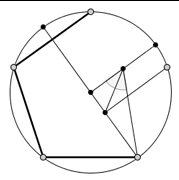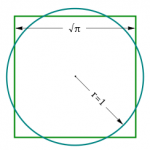By Rumi Salazar, University of New South Wales

There is an intimate connection between an ancient problem posed by the Greeks and a field of mathematics called algebraic number theory. A particular problem that the ancient Greeks were interested in was: What regular polygons could be constructed using only a compass and straightedge (that is, a ruler with no markings)? They could construct triangles, squares, pentagons, and knew at least in principle how to construct a regular polygon with double the number of sides of any given regular polygon. However, they did not know whether it was possible in general to construct any regular polygon.

This problem from antiquity was left unsolved until a (now famous) young mathematician named Carl Friedrich Gauss came along and proved that the 17-gon is constructible, and provided a partial solution to the general problem in the early 1800s. The full solution was completed by another mathematician named Pierre Wantzel in 1837.

We will come back to their solution shortly as we must first introduce the notion of a Fermat prime! In the 1600s, a mathematician and lawyer named Pierre de Fermat studied numbers of the form 2^n+1 (where n=2^k) which are now called Fermat numbers. He noticed that the first five of these numbers, that is, 3, 5, 17, 257, and 65537, are prime numbers. So, he conjectured that all Fermat numbers are prime. Funnily enough, it was shown by Leonhard Euler in 1732 (another famous mathematician), that only the next Fermat number is not prime. It is still not known whether or not there are any other Fermat primes (Fermat numbers that are prime).Having introduced the notion of a Fermat prime, we return to Gauss and Wantzel’s solution to the problem, which is known as the Gauss-Wantzel Theorem. It states: A regular polygon with n sides is constructible if and only if n is a power of 2 multiplied by any number of distinct Fermat primes.

So, despite there only being five known Fermat primes, they interestingly show up in the above theorem! The proof of this theorem involves the theory of field extensions in algebraic number theory. In particular, it turns out that the question of constructible polygons posed by the ancient Greeks boils down to whether or not the solutions of certain types of polynomial equations can be expressed using only the operations of addition, subtraction, multiplication, division, and square roots.

Using the exact same theory of field extensions, one can also show that it is impossible to double the cube, trisect the angle, and square the circle (construct a square with the same area as a circle) using only compass and straightedge constructions!This problem captures, at least in part, the beauty of mathematics. The notion of mathematical beauty deserves many books in its own right, but specifically what I mean is the astounding fact that some easily understood problems in one area of mathematics can often turn out to have very deep solutions in a seemingly disparate area of mathematics. It is the connections between different fields of mathematics that constitute mathematical beauty.

For those interested in further reading, I have provided the following links:

Rumi Salazar was a recipient of a 2018/19 AMSI Vacation Research Scholarship.